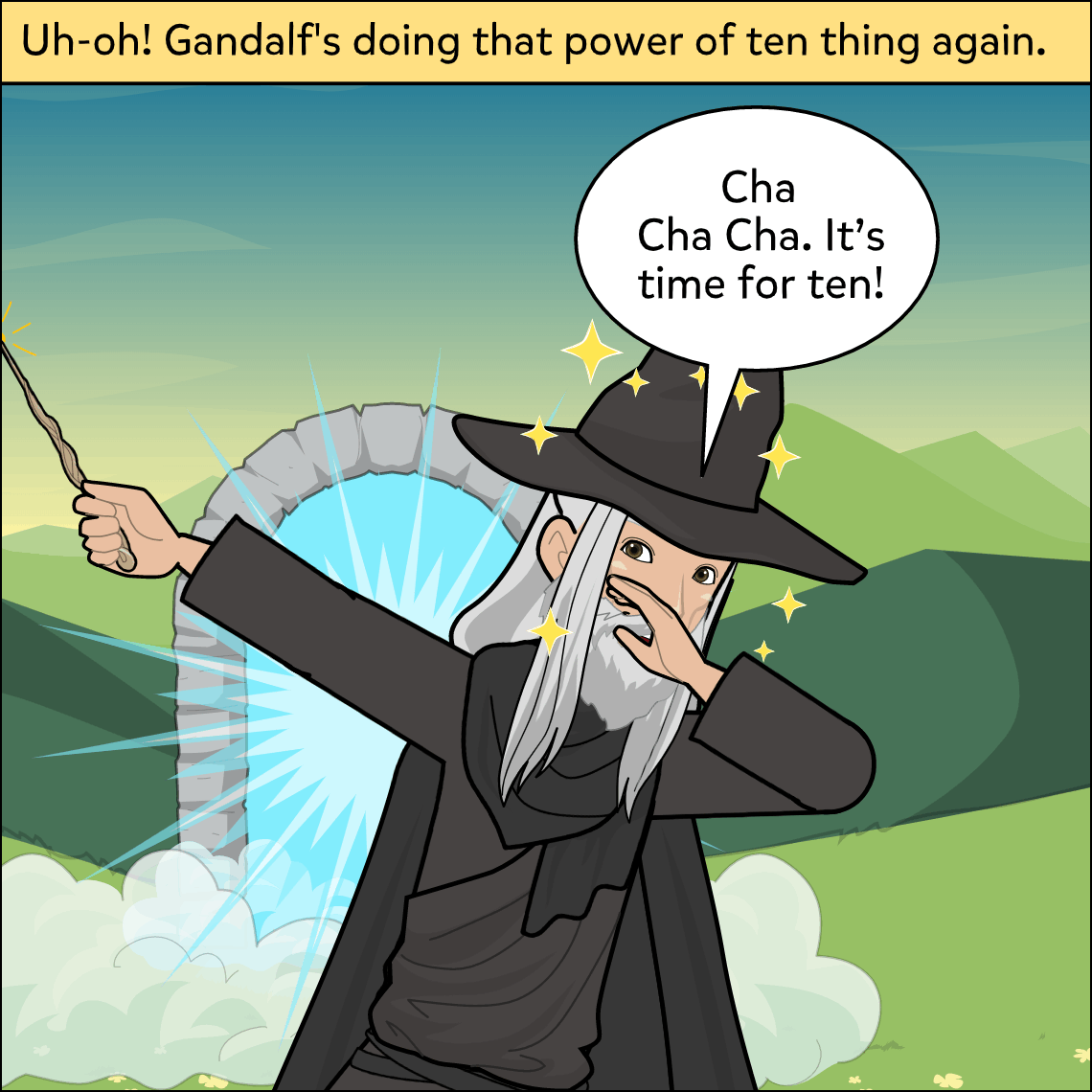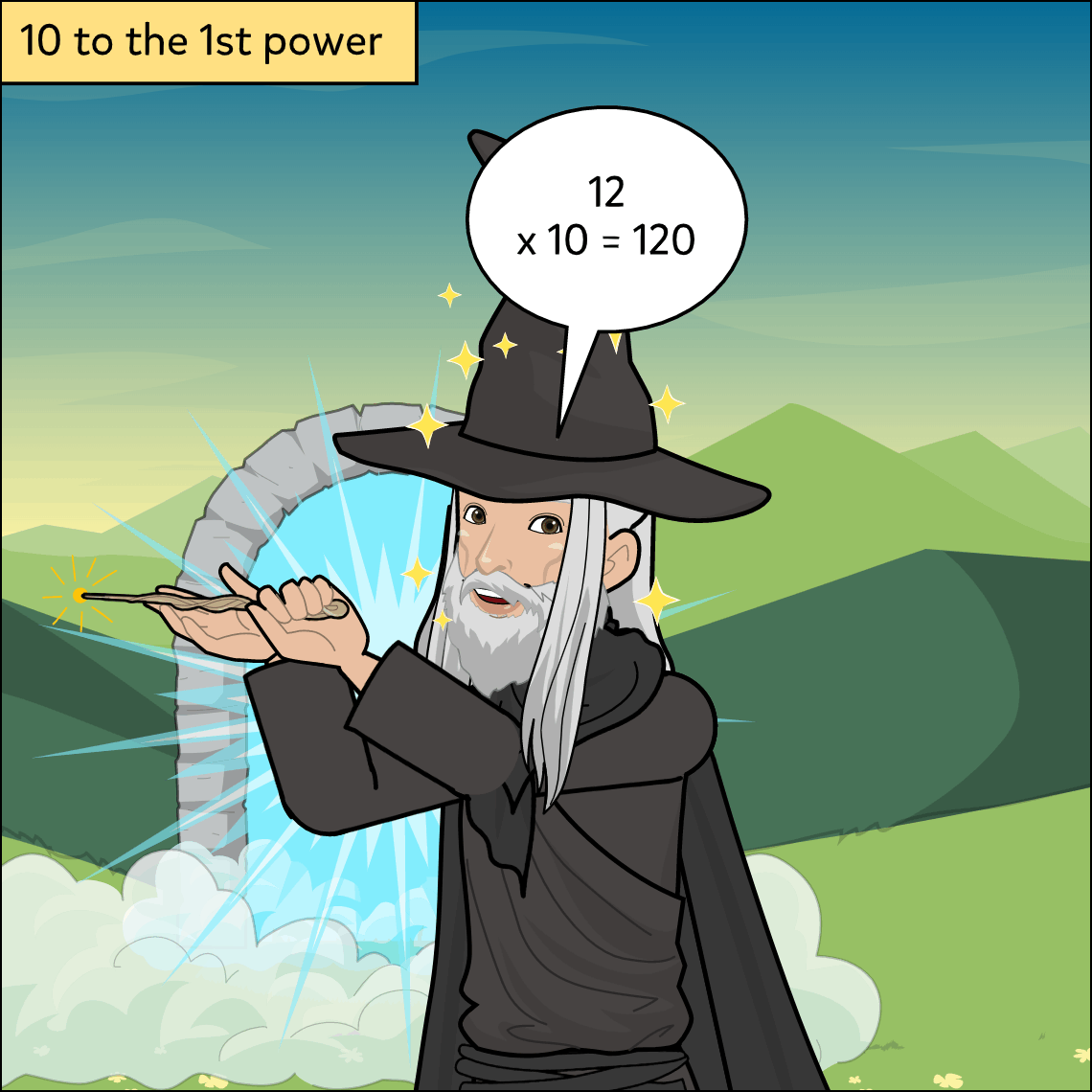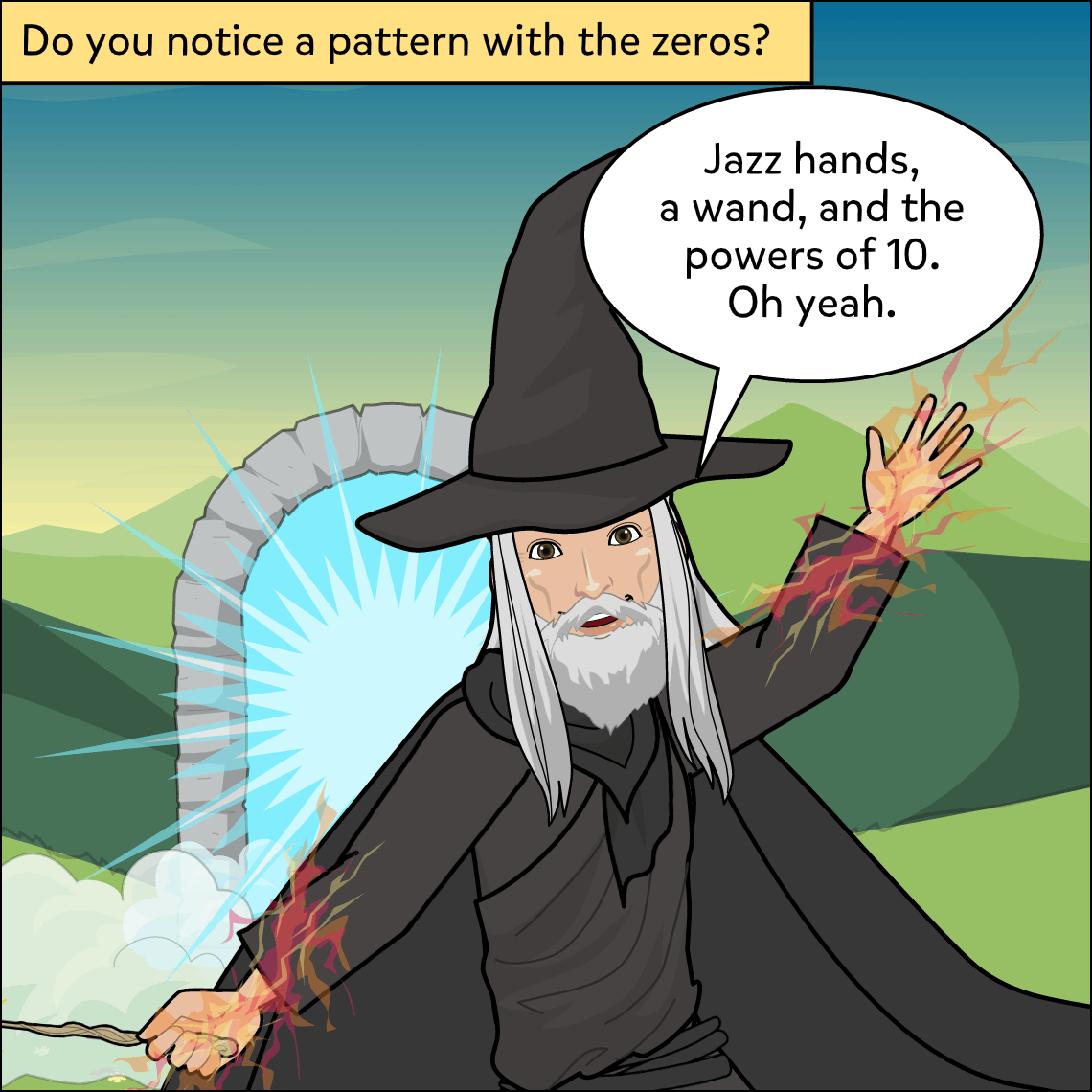Subject: Math

Lesson Length: 30 - 45 mins

Topic: Numbers & Operations in Base Ten- Place Value in Multiplication

Objective: Explanation

Standards: CCSS.MATH.CONTENT.5.NBT.A.2

Standards:

Explain patterns in the number of zeros of the product when multiplying a number by powers of 10, and explain patterns in the placement of the decimal point when a decimal is multiplied or divided by a power of 10. Use whole number exponents to denote powers of 10.

Brief Description: In this lesson, students will explain the pattern of the number of zeros of the product when multiplying by powers of 10. Students will recognize that powers of 10 are represented as 10 with an exponent.

Know Before You Start: This can be used as an introductory lesson to multiplying by powers of 10.Hook: Read and discuss the sample comic. As each number is multiplied, what pattern do they notice?  Discuss what powers of 10 are and how they are expressed with exponents.

Activity:

• Have students choose a number between 1 and 9 and multiply that number by 4 powers of 10 (101, 102, 103, 104).
• Remind students that they can find the product by adding the same number of zeros as the power of ten. (For example, 14 x 102  can be solved by adding two zeros to the number they are multiplying by).
• Have students compare their products with a partner.

Extension if time allows:

• Using the sample comic as a guide, have students create a 6 frame comic showing a number of their choosing multiplied by 5 powers of 10, one in each frame (101, 102, 103, 104, 105).
• Students will explain how the pattern of zeros works in the 6th frame.

Closure: Discuss how many zeros would be required for 1010 based on the way the pattern of zeros works.

Differentiation:

• Allow students to use speech-to-text feature in their comic as needed.
• Provide sentence frames for students on the 6th frame.
• Provide manipulatives and visuals as needed.

Resources: Comic to print or display: Comic 1.